# Tony Marston - 5th December 2001

The term 'Calculation Engine' is used here to describe a method whereby calculations can be performed using formulae that are obtained from the database instead of being hard-wired into any program code. This means that adjustments to the formulae can be made very quickly using a normal data entry screen instead of requiring a programmer to go into the source code, then amend, compile and release it. As you know this is a very time-consuming and expensive process. The Calculation Engine needs to be compiled and released only once - all subsequent customisation of the formulae is simply a matter of changing data. The only arithmetic statements that are hard-wired into the Calculation Engine are:

```ADD something TO something
SUBTRACT something FROM something
MULTIPLY something BY something
DIVIDE something BY something
```

where the various `something` elements are identified at run time from the contents of the FORMULA-LINE table.

# Background

Several years ago I was asked to help a sister company to build a payroll package in UNIFACE. This was to replace the several different versions they had which ran on different platforms with a single 'open' solution. As well as giving them some guidance in how to develop applications in UNIFACE I was asked if I could design some advanced features which did not exist in any of their existing systems. One of these was a flexible organisational hierarchy which is documented in my article A Flexible Tree Structure. Another was a Calculation Engine, which is the subject of this paper.

One of the problems with selling a standard payroll package to lots of different customers is that some customers have requirements, such as the calculation of Profit Related Pay (PRP), which are totally unique. It was getting a bit tedious to keep releasing software with the different calculations hard-wired into the code, so a more flexible solution was sought.

One of the salesmen had seen a rival package where some formulae could be user-defined in SQL statements, but as that package was tied to a particular database it was not really 'open' enough. What I wanted to do was produce a solution that was 100% pure UNIFACE so that it would be truly portable across any platform and any database. I ended up with a solution where not just the PRP but the entire payslip could be calculated using formulae that were obtained from the database. These formulae are accessible via normal data entry screens, thus can be maintained by any user who is familiar with the command syntax. A truly flexible solution that should be able to cope with any situation without requiring the intervention of an expensive programmer.

I should mention that up until this point I had never worked on any payroll system whatsoever, and when I asked for the specific requirements for some of the calculations all I got were copies of one of their User Guides and some database layouts. This meant that in some cases I had to make educated guesses based on nothing more than the fact that I had been on the receiving end of payslips for many years. Some of you may spot errors in some of my formulae, but you should also be quick to realise that any mistakes can be corrected very easily without having to change any program code. Well, that's my excuse and I'm sticking to it.

# The Database Design

Figure 1 - Entity-Relationship Diagram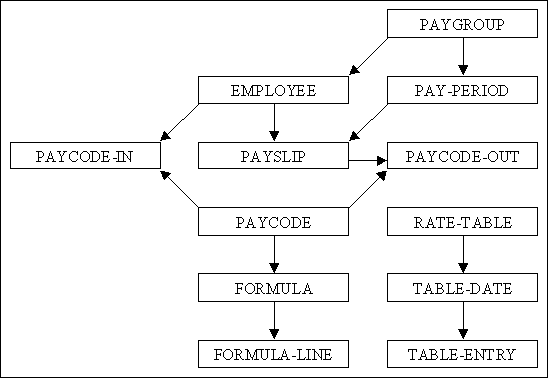In this E-R diagram the arrowhead identifies the MANY in each ONE-to-MANY relationship.

Table 1 - Database Table Contents
table name contents
PAYGROUP PAYGROUP_ID, paygroup_desc
EMPLOYEE EMPLOYEE_ID, paygroup_id, employee_name, blah, blah, blah
PAYCODE-IN EMPLOYEE_ID, PAYCODE_ID, DATE-SEQ, input-hours, input-value, input-percent, start-date, end-date, node_id
PAY-PERIOD PAYGROUP-ID, PAY-YEAR, PAY-PERIOD, start-date, end-date, pay-date
PAYSLIP EMPLOYEE-ID, PAYSLIP-ID, paygroup-id, pay-year, pay-period
PAYCODE-OUT EMPLOYEE-ID, PAYSLIP-ID, PAYCODE-ID, output-value
PAYCODE PAYCODE-ID, sort-seq, paycode-desc, paycode-type, allow-hours, allow-value, allow-percent, allow-node
FORMULA PAYCODE-ID, DATE-SEQ, start-date, end-date
FORMULA-LINE PAYCODE-ID, DATE-SEQ, LINE-NO, formula-line
RATE-TABLE TABLE-ID, table-desc
TABLE-DATE TABLE-ID, DATE-SEQ, start-date, end-date
TABLE-ENTRY TABLE-ID, DATE-SEQ, ENTRY-SEQ, rate-band, rate-amount

I have simplified the contents here in order to remove unnecessary complications. The primary key fields are shown in upper case, and the foreign keys should be obvious.

## Comments on the Database Design

As I have stated previously my only contact with any sort of payroll system before this point was nothing more than receiving a payslip at the end of each month, so I started off by studying a payslip for inspiration. I noticed immediately that each amount had a unique identifier, such as TAX-CODE, SALARY, TAX-PAID, NET-PAY and so on, so this identifier became my PAYCODE-ID. Each PAYCODE also has its own method for producing an amount, either by direct input or a specific calculation, so these methods became my FORMULAE. Each FORMULA is maintained via a standard UNIFACE form using plain English statements that are described in section The Command Syntax for defining a Formula which is defined below.

 PAYCODE-ID A user-defined string that defines each element in a payslip. PAYCODE-DESC A description for this PAYCODE entry. SORT-SEQ Defines the order in which the various formulae are processed. PAYCODE-TYPE Either 'input allowed' or 'output only'. Only those with 'input allowed', such as TAX-CODE and SALARY, can appear on the PAYCODE-IN table. ALLOW-VALUE Options are: NONE, Numbers only, Letters only, or Both. If PAYCODE-TYPE is 'output only' then this can only be 'NONE'. This determines the format of the INPUT-VALUE field on the PAYCODE-IN table. For example, TAX-CODE is a mixture of numbers and letters, while SALARY must be numbers only. ALLOW-HOURS BOOLEAN: if YES then the PAYCODE-IN occurrence can contain a number of hours. This can be used to identify how many hours were worked on a particular task, either as a calculation of wages or for charging that amount to the relevant nominal code in the accounting system. ALLOW-PERCENT BOOLEAN: if YES then the PAYCODE-IN occurrence can contain a percentage value. This can be used to determine the amount which the employee pays into a pension scheme, for example. ALLOW-NODE BOOLEAN: if YES then the PAYCODE-IN occurrence can contain a NODE-ID. This is used to identify an entry in the structure hierarchy, such as project code or department, to which to which the amount is to be charged. This structure hierarchy is described in a separate paper called A Flexible Tree Structure.

Figure 2 - Screenshot of PAYCODE-IN (summary)The PAYCODE-IN table contains the variables that are required for each individual EMPLOYEE, such as TAX-CODE, SALARY, etc. Each entry has a start and end date so that a full history of all changes can be maintained. This is implemented using a standard design technique which I have documented in a separate paper called Keeping a history of changes by date. This means that when a payroll calculation is performed for a specific date then only those values that apply to that date are included.

For some employees the data here can be fairly static, but for others it may involve the input of a weekly timesheet to record the number of hours worked on a particular task, either for the calculation of wages or to identify to whom the amount is to be charged.

Figure 2 shows all the current input values that apply to this particular employee. Figure 3 shows the complete history for one of those paycodes.

Figure 3 - Screenshot of PAYCODE-IN (detailed history)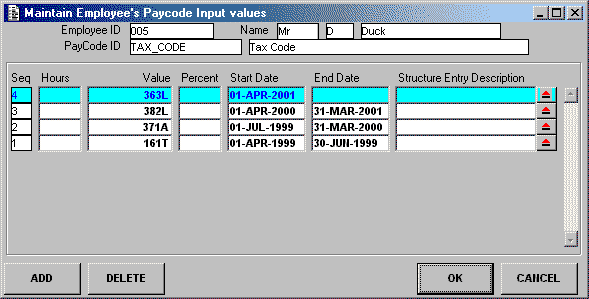Figure 3 shows the history of one particular PAYCODE-ID showing how the value has changed over a period of time. Entries can be input with a future start date and will not be processed until that date is specified for the calculation.

Figure 4 - Screenshot of RATE-TABLE entriesInstead of having a separate set of database tables for each different set of rates, e.g. tax rates and national insurance rates, I decided to maintain them all on one set of tables. Each different set of rates is identified by its own TABLE-ID, as shown in Figure 4.

Figure 5 - Screenshot of TABLE-DATE and TABLE-ENTRY entries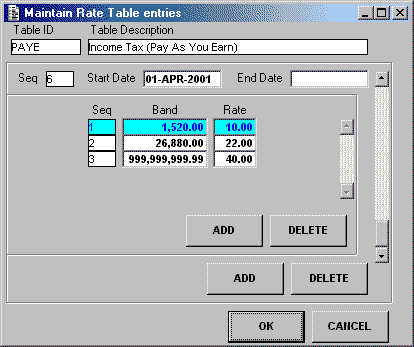As is usual there can be different sets of values for each TABLE-ID for different time periods. Figure 5 shows the PAYE rates that came into force on April 1st 2001. This shows that the first ?1,520 will be taxed at 10%, the next ?26,880 will be taxed at 22%, and the remainder will be taxed at 40%. Entries can be input with a future start date and will not be processed until that date is specified for the calculation.

Figure 6 - Screenshot of the FORMULA and FORMULA-LINE tables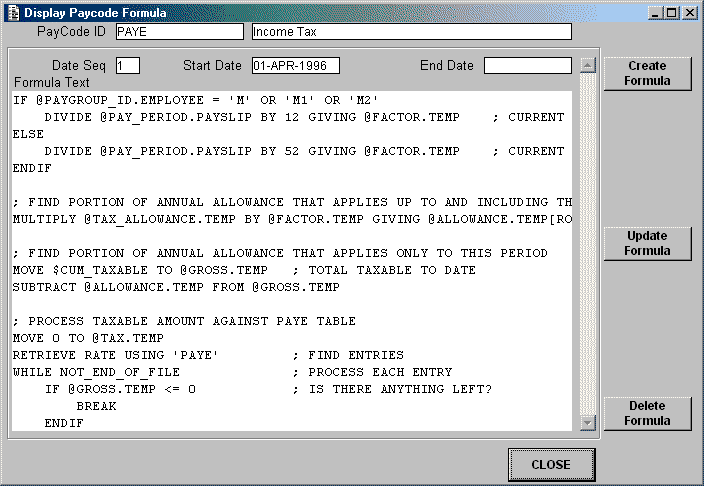Figure 6 shows a screen displaying the formula for one of the paycodes. Notice again that the formula for each paycode may change over a period of time, but each change is held with its own start and end dates. When a calculation run is processed for a specific date only the version which applies to that date will be used.

Although each line of the formula is held on a separate database occurrence these are all merged into a single multi-line field for display and maintenance purposes. Large formulae can be better accessed by using the ZOOM function on the field. The grammar used to define each formula is described in section The Command Syntax for defining a Formula.

The PAYGROUP table is simply a method of breaking down the list of employees into different processing groups, such as monthly paid, weekly paid, directors, etc. Each payslip run starts off by selecting a PAYGROUP and a PAY-PERIOD, then all the employees in that group are processed one at a time using the variables that apply to the specified PAY-PERIOD.

The PAY-PERIOD table is used to define the applicable dates for the next payslip calculation run for employees in the PAYGROUP.

The PAYSLIP table is used to record whenever a payslip is generated for an employee. The PAYSLIP-ID starts at 1 for each employee and is incremented by 1 for each new payslip.

Figure 7 - Screenshot of the PAYCODE-OUT table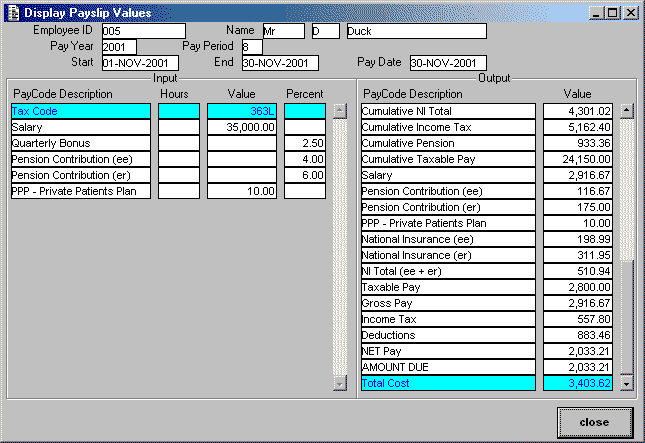The PAYCODE-OUT table contains the output from all payslip calculations. Note that not all of the entries will actually appear on the printed payslip. Some values are required so that they can be carried forward into the next calculation, other values are required because they must be entered into an external accounting system.

None of the cumulative values, such as GROSS-PAY-TO-DATE or TAXABLE-PAY-TO-DATE are held on the EMPLOYEE record, they are all held here. Whenever any calculation requires one of these cumulative amounts it obtains it by looking at the contents of the previous payslip. This is easily identified as the PAYSLIP-ID of the previous payslip is always 1 less than the PAYSLIP-ID of the current payslip.

# The Command Syntax for defining a Formula

I make absolutely no apologies for the fact that my command syntax has an extremely close resemblance to those found in the COBOL language. It was, after all, my primary language for 16 years before I switched to UNIFACE. A colleague tried to suggest that I use something similar to C++ notation, but I prefer using plain English, not gobbledegook.

## Summary of Commands

Each line of a formula should consist of a single statement terminated by a carriage return. For a statement to be valid it must match one of the following:

```MOVE     <input>        TO   <output>
ADD      <input>        TO   <input>        GIVING <output>
SUBTRACT <input>        FROM <input-output>
SUBTRACT <input>        FROM <input>        GIVING <output>
MULTIPLY <input-output> BY   <input>
MULTIPLY <input>        BY   <input>        GIVING <output>
DIVIDE   <input-output> BY   <input>
DIVIDE   <input>        BY   <input>        GIVING <output>

RETRIEVE <table_name> USING <table_id>

IF <logical expression>
statement
....
ELSE
statement
....
ENDIF

WHILE <logical expression>
statement
....
ENDWHILE

REPEAT
statement
....
UNTIL <logical expression>

BREAK - terminate the current WHILE-ENDWHILE or REPEAT-UNTIL loop.

STOP - stop further processing of the current formula
```

Blank lines are allowed to separate logical blocks of code

A ';' (semi-colon) denotes the start of comments, which will be ignored when processing the formula. Comments are allowed to follow statements on the same line, or can be on lines of their own.

Note that it is not necessary for a PAYCODE to have a FORMULA which provides the value for that PAYCODE. It is possible for values to be set during the processing of formulae for other PAYCODEs.

## Input fields

`<input>` defines an input source, which can be one of the following:

• a literal number, with an optional decimal point (eg: 12, 10.4)
• a literal number followed by a percentage sign (eg: 25%, 10.4%) (see NOTE1 below)
• a literal string enclosed in single quotes (eg: 'K')

• INPUT_VALUE - uses the value (numeric part) from the PAYCODE_IN entry
• INPUT_VALUE(A) - uses the value (alphabetic part) from the PAYCODE_IN entry
• INPUT_HOURS - uses the HOURS value from the PAYCODE_IN entry
• INPUT_PERCENT - uses the PERCENT value from the PAYCODE_IN entry (see NOTE1 below)

• \$PAYCODE_ID - uses the current value for the named PAYCODE_ID within the calculation for the current Employee (e.g. \$SALARY, \$NET_PAY)
• \$PAYCODE_ID(B/F) - as above, but brought forward from the PREVIOUS payroll period (where PAYSLIP_ID is 1 less than the current PAYSLIP_ID)

• @item.table - uses the current value for this database item.
• The 'table' must be one of 'EMPLOYEE', 'PAYSLIP' or 'RATE' (i.e. any table which is included in the structure of the calculation component).
• The 'item' must exist as a fieldname on the specified database table.

• @item.TEMP - temporary work area for holding intermediate results. In this case 'item' can be any name.

NOTE1: Percentage values will automatically be divided by 100 before being used in any calculation.

## Output fields

`<output>` defines an output destination, which must be:-

• \$PAYCODE-ID - the identity of an entry on the PAYCODE table, such as `\$NET_PAY`. If the value is non-zero at the end of the calculation a record will be added to the PAYCODE_OUT table.
• @item.TEMP - temporary work area for holding intermediate results. In this case 'item' can be any name as these values are never written out to the database.

## Rounding

`<output>` values can be rounded UP or DOWN as required:

• `<output>[ROUND,n]` - the value in `<output>` will be rounded UP
• `<output>[TRUNC,n]` - the value in `<output>` will be rounded DOWN

The digit after the `ROUND` or `TRUNC` specification identifies where the rounding is to take place:

• A positive value (1 to 9) indicates the number of digits after the decimal point.
• A negative value (-1 to -9) indicates the number of digits before the decimal point.
• A zero value indicates that rounding will take place at the decimal point.

## IF-ELSE-ENDIF processing

Either of the following constructs is valid:

```IF <logical expression>
statement
....
ELSE
statement
....
ENDIF
```

or

```IF <logical expression>
statement
....
ENDIF
```

An `IF` statement may be nested inside another `IF` or `LOOP` statement to any number of levels.

```IF <logical expression>
IF <logical expression>
statement
....
ENDIF
ENDIF
```

## WHILE-ENDWHILE and REPEAT-UNTIL loops

The `WHILE-ENDWHILE` loop will execute all enclosed statements until `<logical expression>` is FALSE, and will evaluate the expression before each iteration. If `<logical expression>` is FALSE to begin with then the statements are never executed.

The `REPEAT-UNTIL` loop will execute all enclosed statements until `<logical expression>` is TRUE, and will evaluate the expression after each iteration. If `<logical expression>` is TRUE to begin with then the statements are executed only once.

`BREAK` will terminate the current `WHILE-ENDWHILE` or `REPEAT-UNTIL` loop.

An `LOOP` statement may be nested inside another `IF` or `LOOP` statement to any number of levels.

## Logical expressions

`<logical expression>` is in the format `<input1> RELOP <input2>`, where `RELOP` is the Relational Operator which is used to compare the two `<input>` values. It must be one of the following:

```=  (equal to)
!= (not equal to)
<  (less than)
<= (less than or equal to)
>  (greater than)
>= (greater than or equal to)
```

If RELOP is '=' (equal to) then up to 4 choices are allowed, as in:

```IF    @PAY_PERIOD.PAYSLIP = 3 or 6 or 9 or 12
WHILE @PAY_PERIOD.PAYSLIP = 3 or 6 or 9 or 12
UNTIL @PAY_PERIOD.PAYSLIP = 3 or 6 or 9 or 12
```

or

```IF INPUT_VALUE(A) = 'A' OR 'B' OR 'C' OR 'D'
```

Following a `RETRIEVE` or `READ` command the following conditions are set to either TRUE or FALSE as appropriate:

```END_OF_FILE     (no more records exist)
NOT_END_OF_FILE (record exists)
```

These two expressions can be used in statements as follows:

```IF (NOT_)END_OF_FILE
UNTIL END_OF_FILE
WHILE NOT_END_OF_FILE
```

## TABLE processing

```RETRIEVE <table_name> USING <table_id>
```

`<table-name>` identifies the database table to be processed. Currently the only database table which can be accessed this way is the RATE table.

`<table_id>` identifies the primary key value for table entry, which must exist on that table.

`<record_number>` is a numeric literal or an `<input>` value which contains a number.

`RETRIEVE` will establish a hitlist for a set of database records, making them available for subsequent `READ` operations. If the `RETRIEVE` is successful it will perform an automatic `READ` of the first record, and will set `END_OF_FILE` to FALSE (and `NOT_END_OF_FILE` to TRUE).

Subsequent database records can be obtained with the `READ` command. If the `USING <record_number>` argument is used this will obtain the record with that occurrence number in the hitlist, otherwise the next available record will become the current record.

If there is no next record, or `<record_number>` is invalid, `END_OF_FILE` will be set to TRUE (and `NOT_END_OF_FILE` to FALSE).

# Example formulae

## Initialising an accumulator

```IF @PAY_PERIOD.PAYSLIP = 1
MOVE 0 TO \$CUM_BASIC
ELSE
MOVE \$CUM_BASIC(B/F) TO \$CUM_BASIC
ENDIF
```

Here the value for `\$CUM_BASIC` is set to zero at the beginning of each year, and in other periods the value from the previous payslip is brought forward.

## Using INPUT-VALUE from PAYCODE-IN

```; STORE THIS FOR BONUS CALCULATION
MOVE INPUT_VALUE TO @SALARY.TEMP
; GET MONTHLY AMOUNT FROM ANNUAL SALARY
DIVIDE INPUT_VALUE BY 12 GIVING @HOLD.TEMP[ROUND,2]
;
MOVE @HOLD.TEMP TO \$BASIC
MOVE @HOLD.TEMP TO \$GROSS
MOVE @HOLD.TEMP TO \$NET
MOVE @HOLD.TEMP TO \$TAXABLE
MOVE @HOLD.TEMP TO @PENSIONABLE.TEMP
```

This divides the annual salary by 12 to get the monthly amount (basic pay). Notice how other values used in the calculation process are affected at this point.

Notice that the amount of salary which is pensionable is held as a temporary variable (`@PENSIONABLE.TEMP`) instead of being output to PAYCODE-OUT (`\$PENSIONABLE`) as it does not require to be printed on the payslip, accumulated for future payslips, or exported to an external accounting system.

## Using INPUT-VALUE(A) from PAYCODE-IN

```IF INPUT_VALUE(A) = 'E' OR 'K'
MOVE 0 TO @TAX_ALLOWANCE.TEMP
STOP
ENDIF
MULTIPLY INPUT_VALUE BY 10 GIVING @TAX_ALLOWANCE.TEMP
```

If the TAX-CODE is input as '363L' then:

• `INPUT_VALUE(A)` would provide 'L'
• `INPUT_VALUE` would provide '363'

## Using INPUT-PERCENT from PAYCODE-IN

```MULTIPLY @PENSIONABLE.TEMP BY INPUT_PERCENT GIVING @HOLD.TEMP[ROUND,2]
IF INPUT_VALUE > @HOLD.TEMP
MOVE INPUT_VALUE TO @HOLD.TEMP
ENDIF
;
MOVE     @HOLD.TEMP TO   \$PENSION_1
SUBTRACT @HOLD.TEMP FROM \$TAXABLE
SUBTRACT @HOLD.TEMP FROM \$NET
```

This is where an employee's pension contributions are initially expressed as a percentage of basic pay, but can be replaced by a specified amount if this is a higher value.

## Using INPUT-HOURS from PAYCODE-IN

```MULTIPLY INPUT_HOURS BY INPUT_VALUE GIVING @HOLD.TEMP

```

This is where the PAYCODE-IN record contains both a number of hours and an hourly rate.

## IF-ELSE-ENDIF processing

```; ONLY PROCESS AT THE END OF EACH QUARTER
IF @PAY_PERIOD.PAYSLIP = 3 OR 6 OR 9 OR 12
IF INPUT_VALUE > 0
; USE SPECIFIED VALUE
MOVE INPUT_VALUE TO @HOLD.TEMP
ELSE
; USE A PERCENTAGE OF ANNUAL SALARY
MULTIPLY @SALARY.TEMP BY INPUT_PERCENT GIVING @HOLD.TEMP[ROUND,2]
ENDIF
MOVE @HOLD.TEMP TO \$BONUS_Q
ENDIF
```

This calculates a quarterly bonus, which is why it is not included in every period. The amount can either be a percentage of the annual salary or a specific amount.

## LOOP and TABLE processing

The basic code looks something like this:

```RETRIEVE RATE USING 'PAYE'         ; FIND ENTRIES
WHILE NOT_END_OF_FILE              ; PROCESS EACH ENTRY
....
ENDWHILE

or

RETRIEVE RATE USING 'PAY-BAND'     ; FIND ENTRIES
```

When part of a complete formula it can look something like this:

```IF @PAYGROUP_ID.EMPLOYEE = 'M' OR 'M1' OR 'M2'
DIVIDE @PAY_PERIOD.PAYSLIP BY 12 GIVING @FACTOR.TEMP    ; CURRENT MONTH
ELSE
DIVIDE @PAY_PERIOD.PAYSLIP BY 52 GIVING @FACTOR.TEMP    ; CURRENT WEEK
ENDIF

; FIND PORTION OF ANNUAL ALLOWANCE THAT APPLIES UP TO AND INCLUDING THIS PERIOD
MULTIPLY @TAX_ALLOWANCE.TEMP BY @FACTOR.TEMP GIVING @ALLOWANCE.TEMP[ROUND,2]

; SUBTRACT FROM CUMULATIVE TAXABLE TO GIVE TAXABLE AMOUNT FOR THIS PERIOD
MOVE \$CUM_TAXABLE TO @GROSS.TEMP   ; TOTAL TAXABLE TO DATE
SUBTRACT @ALLOWANCE.TEMP FROM @GROSS.TEMP

; PROCESS TAXABLE AMOUNT AGAINST PAYE TABLE
MOVE 0 TO @TAX.TEMP
RETRIEVE RATE USING 'PAYE'         ; FIND ENTRIES
WHILE NOT_END_OF_FILE              ; PROCESS EACH ENTRY
IF @GROSS.TEMP <= 0            ; IS THERE ANYTHING LEFT?
BREAK
ENDIF
; FIND PORTION OF TAX BAND THAT APPLIES UP TO AND INCLUDING THIS PERIOD
MULTIPLY @RATE_BAND.RATE BY @FACTOR.TEMP GIVING @BAND.TEMP[ROUND,2]
IF @GROSS.TEMP < @BAND.TEMP
MOVE @GROSS.TEMP TO @BAND.TEMP
ENDIF
SUBTRACT @BAND.TEMP FROM @GROSS.TEMP
MULTIPLY @BAND.TEMP BY @RATE_AMOUNT.RATE GIVING @HOLD.TEMP[ROUND,2]
ENDWHILE

SUBTRACT \$CUM_PAYE FROM @TAX.TEMP GIVING @HOLD.TEMP ; SUBTRACT TAX ALREADY PAID

MOVE     @HOLD.TEMP TO   \$PAYE
SUBTRACT @HOLD.TEMP FROM \$NET
```

This formula uses the rates from the 'PAYE' table to calculate the amount of income tax that is due this period. Each occurrence from the RATE table contains a percentage rate plus a maximum amount of income which is to be taxed at this rate. Note that the tax allowance and tax rates are expressed as annual amounts, but I have to adjust these to provide amounts which apply up to and including the current period.

# The Calculation process

The calculation module is activated with the parameters EMPLOYEE-ID, PAY-YEAR and PAY-PERIOD. It then performs the following steps:

1. Retrieve the EMPLOYEE record. Check that the employee's start and end dates fall within the specified pay period. If not then generate an error message.
2. If a payslip for this period already exists then delete it.
3. Check that there are entries on the PAYCODE-IN table which fall within the specified pay period. If not then generate an error message.
4. Retrieve all PAYCODE entries and all associated FORMULA-LINES which fall within the specified pay period. For the second and subsequent employees in the same calculation run these occurrences are not retrieved again, instead the output values are initialised.
5. Initialise all temporary variables (@item.TEMP).
6. Process each PAYCODE entry in the order specified by SORT-SEQ:
1. If the PAYCODE-TYPE is 'input allowed' then process the formula once for each occurrence on PAYCODE-IN.
2. For other values of PAYCODE-TYPE process the formula just once.
7. When all PAYCODE entries have been processed then create an entry on PAYCODE-OUT for each occurrence of PAYCODE which has a non-zero amount for output value.

With this design it is possible to recalculate an employee's payslip as many times as I like without having to undo the results of the previous calculation. This is because there are no cumulative totals on the EMPLOYEE record which have to be rolled back prior to the next calculation. I noticed in the User Guide for the old payroll system that a payslip calculation could not be redone without performing an UNPAY operation first, and that it was not possible to go back further than the last payslip. There is no such restriction in my design. All the values generated by a payslip calculation, including cumulative totals, are held on the PAYCODE-OUT table, so obtaining one of these values from the previous payslip requires no more than a single lookup using a primary key that is easy to generate - the value for PAYSLIP-ID for the previous payslip is always 1 less than the value for the current payslip.

This ability was also great for testing purposes as I could take all my payslips for a whole year, including changes in tax code and salary, and run through them as many times as I liked until I got the formulae perfected.

# The Audit Trail

While I was testing my code it quickly became apparent that it was too tedious to step through each iteration with the debugger, so I modified it to include the ability to output a processing trace or audit trail. This uses the `PUTMESS` command to append lines of text to the message frame. Each line can be one of the following:

a) At the start of each PAYCODE:

`==> ********** PAYCODE_ID = XXXX`

where 'XXXX' is the identity of the PAYCODE being processed.

b) At the start of each FORMULA-LINE:

`(n) text`

where `(n)` is the number of the FORMULA-LINE being processed
and `'text'` is the contents of that FORMULA-LINE.

c) After a FORMULA-LINE that contains input or output values:

`==> text`

where 'text' is the line after the `<input>` and `<output>` names have been replaced by their actual values.

d) After a FORMULA-LINE that contains conditional processing:

```==> condition TRUE
or
==> condition FALSE - skip following lines
or
==> condition TRUE - resume processing
or
==> BREAK encountered - skip until following ENDWHILE/UNTIL
```

e) After a FORMULA-LINE that contains a RETRIEVE or READ statement:

```==> curocc= n
or
==> END_OF_FILE= TRUE
```

This is an example of the trace produced while processing the formula shown in LOOP and TABLE processing.

```==> ********** PAYCODE_ID = PAYE
(1) IF @PAYGROUP_ID.EMPLOYEE = 'M' OR 'M1' OR 'M2'
==> IF 'M' = 'M' OR 'M1' OR 'M2'
==> condition TRUE
(2)     DIVIDE @PAY_PERIOD.PAYSLIP BY 12 GIVING @FACTOR.TEMP    ; CURRENT MONTH
==>     DIVIDE 8 BY 12 GIVING 0.666666666666666666666666666666666666666
(3) ELSE
==> condition FALSE - skip following lines
(5) ENDIF
==> condition TRUE - resume processing
(8) MULTIPLY @TAX_ALLOWANCE.TEMP BY @FACTOR.TEMP GIVING @ALLOWANCE.TEMP[ROUND,2]
==> MULTIPLY 3630 BY 0.666666666666666666666666666666666666666 GIVING 2420
(11) MOVE \$CUM_TAXABLE TO @GROSS.TEMP   ; TOTAL TAXABLE TO DATE
==>  MOVE 24150 TO @GROSS.TEMP
(12) SUBTRACT @ALLOWANCE.TEMP FROM @GROSS.TEMP
==>  SUBTRACT 2420 FROM 24150 GIVING 21730
(15) MOVE 0 TO @TAX.TEMP
==>  MOVE 0 TO @TAX.TEMP
(16) RETRIEVE RATE USING 'PAYE'         ; FIND ENTRIES
==> curocc= 1
(17) WHILE NOT_END_OF_FILE              ; PROCESS EACH ENTRY
==>  WHILE NOT_END_OF_FILE = NOT_END_OF_FILE
==> condition TRUE
(18)     IF @GROSS.TEMP <= 0            ; IS THERE ANYTHING LEFT?
==>      IF 21730 <= 0
==> condition FALSE - skip following lines
(20)     ENDIF
==> condition TRUE - resume processing
(21)     MULTIPLY @RATE_BAND.RATE BY @FACTOR.TEMP GIVING @BAND.TEMP[ROUND,2]
==> MULTIPLY 1520 BY 0.666666666666666666666666666666666666666 GIVING 1013.33
(22)     IF @GROSS.TEMP < @BAND.TEMP
==>      IF 21730 < 1013.33
==> condition FALSE - skip following lines
(24)     ENDIF
==> condition TRUE - resume processing
(25)     SUBTRACT @BAND.TEMP FROM @GROSS.TEMP
==>      SUBTRACT 1013.33 FROM 21730 GIVING 20716.67
(26)     MULTIPLY @BAND.TEMP BY @RATE_AMOUNT.RATE GIVING @HOLD.TEMP[ROUND,2]
==>      MULTIPLY 1013.33 BY 0.1 GIVING 101.33
==>      ADD 101.33 TO 0 GIVING 101.33
==> curocc= 2
(29) ENDWHILE
(17) WHILE NOT_END_OF_FILE              ; PROCESS EACH ENTRY
==>  WHILE NOT_END_OF_FILE = NOT_END_OF_FILE
==> condition TRUE
(18)     IF @GROSS.TEMP <= 0            ; IS THERE ANYTHING LEFT?
==>      IF 20716.67 <= 0
==> condition FALSE - skip following lines
(20)     ENDIF
==> condition TRUE - resume processing
(21)     MULTIPLY @RATE_BAND.RATE BY @FACTOR.TEMP GIVING @BAND.TEMP[ROUND,2]
==>      MULTIPLY 26880 BY 0.666666666666666666666666666666666666666 GIVING 17920
(22)     IF @GROSS.TEMP < @BAND.TEMP
==>      IF 20716.67 < 17920
==> condition FALSE - skip following lines
(24)     ENDIF
==> condition TRUE - resume processing
(25)     SUBTRACT @BAND.TEMP FROM @GROSS.TEMP
==>      SUBTRACT 17920 FROM 20716.67 GIVING 2796.67
(26)     MULTIPLY @BAND.TEMP BY @RATE_AMOUNT.RATE GIVING @HOLD.TEMP[ROUND,2]
==>      MULTIPLY 17920 BY 0.22 GIVING 3942.4
==>      ADD 3942.4 TO 101.33 GIVING 4043.73
==> curocc= 3
(29) ENDWHILE
(17) WHILE NOT_END_OF_FILE              ; PROCESS EACH ENTRY
==>  WHILE NOT_END_OF_FILE = NOT_END_OF_FILE
==> condition TRUE
(18)     IF @GROSS.TEMP <= 0            ; IS THERE ANYTHING LEFT?
==>      IF 2796.67 <= 0
==> condition FALSE - skip following lines
(20)     ENDIF
==> condition TRUE - resume processing
(21)     MULTIPLY @RATE_BAND.RATE BY @FACTOR.TEMP GIVING @BAND.TEMP[ROUND,2]
==>      MULTIPLY 999999999.99 BY 0.666666666666666666666666666666666666666 GIVING 666666666.66
(22)     IF @GROSS.TEMP < @BAND.TEMP
==>      IF 2796.67 < 666666666.66
==> condition TRUE
(23)         MOVE @GROSS.TEMP TO @BAND.TEMP
==>          MOVE 2796.67 TO @BAND.TEMP
(24)     ENDIF
(25)     SUBTRACT @BAND.TEMP FROM @GROSS.TEMP
==>      SUBTRACT 2796.67 FROM 2796.67 GIVING 0
(26)     MULTIPLY @BAND.TEMP BY @RATE_AMOUNT.RATE GIVING @HOLD.TEMP[ROUND,2]
==>      MULTIPLY 2796.67 BY 0.4 GIVING 1118.67
==>      ADD 1118.67 TO 4043.73 GIVING 5162.4
==> END_OF_FILE= TRUE
(29) ENDWHILE
(17) WHILE NOT_END_OF_FILE              ; PROCESS EACH ENTRY
==>  WHILE NOT_END_OF_FILE = END_OF_FILE
==> condition FALSE - skip following lines
(29) ENDWHILE
==> condition TRUE - resume processing
(31) SUBTRACT \$CUM_PAYE FROM @TAX.TEMP GIVING @HOLD.TEMP ; SUBTRACT TAX ALREADY PAID
==>  SUBTRACT 4604.6 FROM 5162.4 GIVING 557.8
(33) MOVE     @HOLD.TEMP TO   \$PAYE
==>  MOVE 557.8 TO \$PAYE
==>  ADD 557.8 TO 4604.6 GIVING 5162.4
(35) SUBTRACT @HOLD.TEMP FROM \$NET
==>  SUBTRACT 557.8 FROM 2591.01 GIVING 2033.21
==>  ADD 557.8 TO 325.66 GIVING 883.46
==> ********** PAYCODE_ID = DEDUCTIONS
```

As you can see this provides a line-by-line trace of all the calculations used in the generation of a payslip, and is therefore of great benefit when checking how a particular result was arrived at.

# Conclusion

Although this particular design was originally put together for a payroll system, it should be possible to include these techniques in any system where customisable calculations are required. The ability to modify calculations via normal data entry screens instead of changing any hard-wired program code means that changes can be applied in a fraction of the time and at a fraction of the cost.

Tony Marston
5th December, 2001

mailto:tony@tonymarston.net
mailto:TonyMarston@hotmail.com
http://www.tonymarston.net